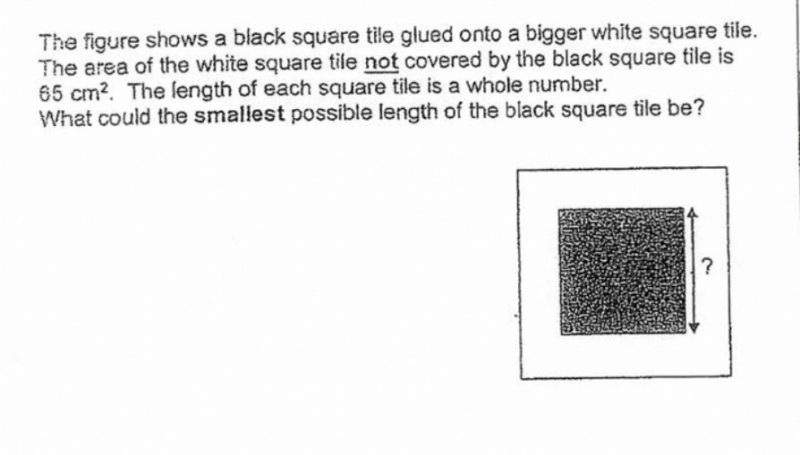# Questionhi, can we get help for this qn? thank you!

1 Answer

# Answer

assume the black square length is a, white is b

not covered is 65, that mean b– a2 = 65, a and b are whole number, a is 4 and b is 9.

note:  b– a2 = (b+a)(b-a), and 65 = 5 x 13 ( not choose 1×65 as we need to smallest)

0 Replies 0 Likes